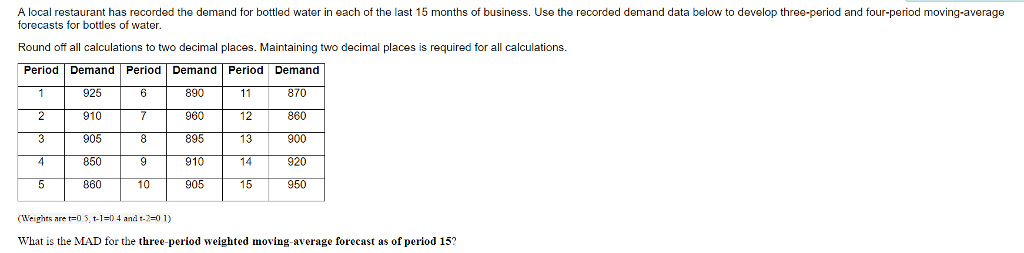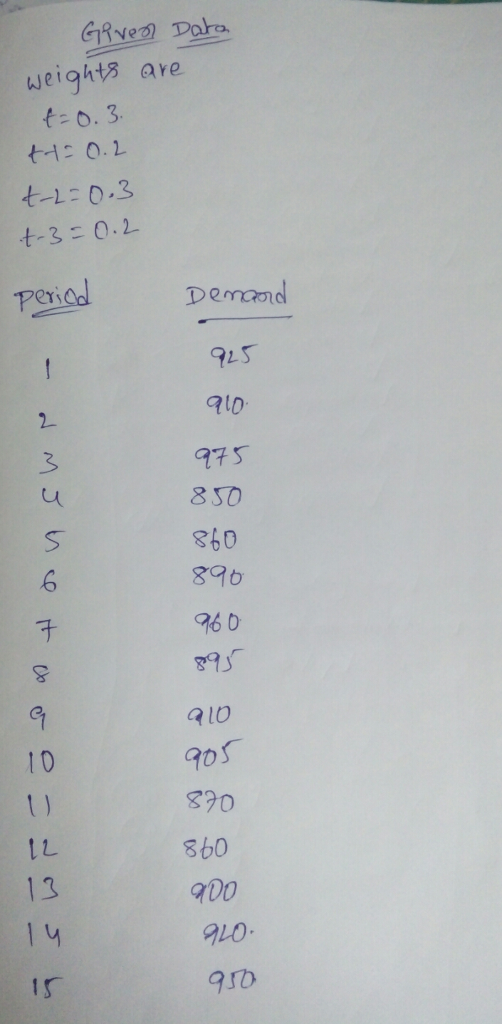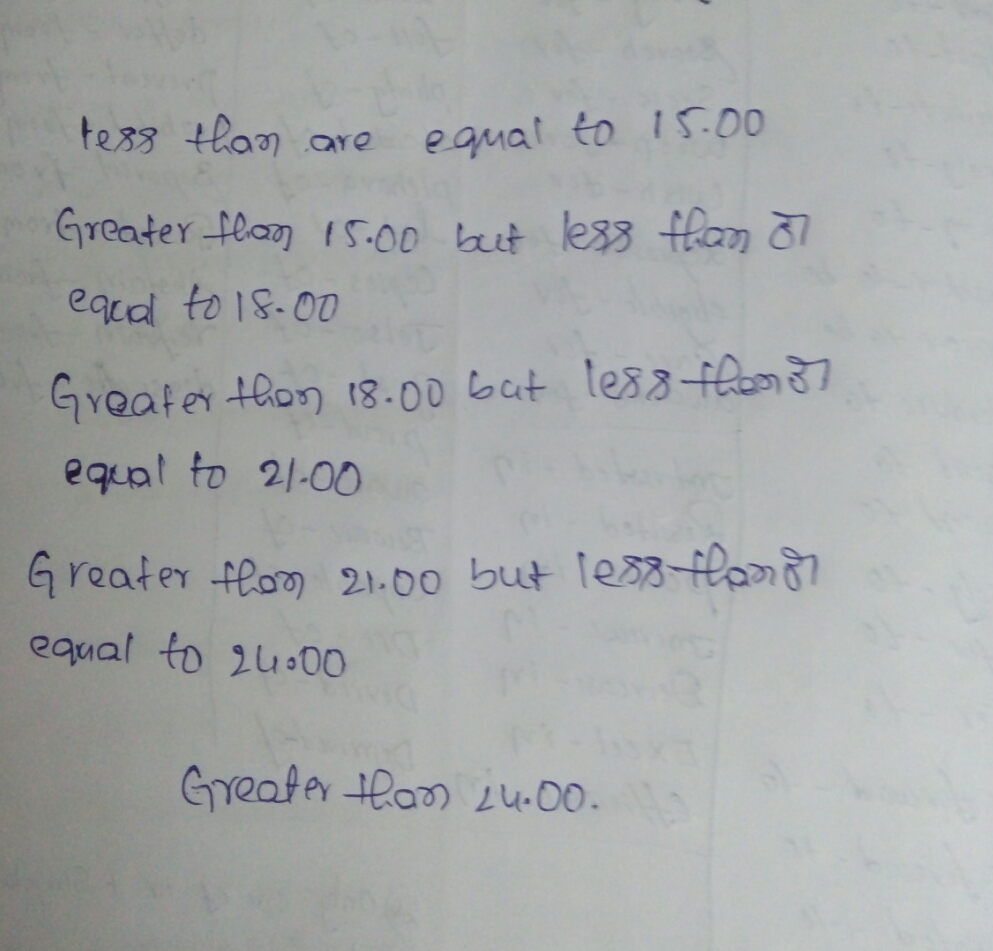# Question & Answer: A local restaurant has recorded the demand for bottled water in each of the last…..

(Weights are t=0.3, t-1=0.2, t-2=0.3 and t-3=0.2)A local restaurant has recorded the demand for bottled water in each of the last 15 months of business. Use the recorded demand data below to develop three-period and four-period moving-average forecasts for bottles of water. Round off all calculations to two decimal places. Maintaining two decimal places is required for all calculations. (Weights are t = 0.5, t -1 = 0.4 and t-2 = 0.1) What is the MAD for the three-period weighted moving-average forecast as of period 15?